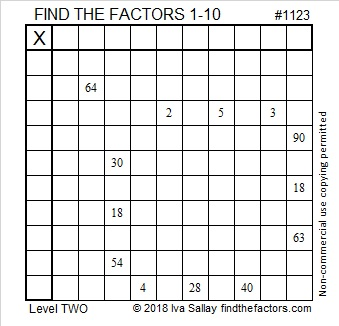# 1123 and Level 2

All the clues in one of the rows of this Level 2 puzzle are prime numbers. The only common factor they have is 1. That fact will get you started with this puzzle which I am sure you can complete if you just give it a try!Print the puzzles or type the solution in this excel file: 10-factors-1121-1133

1123 is also a prime number. Here are some facts about it.

• 1123 is a prime number.
• Prime factorization: 1123 is prime.
• The exponent of prime number 1123 is 1. Adding 1 to that exponent we get (1 + 1) = 2. Therefore 1123 has exactly 2 factors.
• Factors of 1123: 1, 1123
• Factor pairs: 1123 = 1 × 1123
• 1123 has no square factors that allow its square root to be simplified. √1123 ≈ 33.51119

How do we know that 1123 is a prime number? If 1123 were not a prime number, then it would be divisible by at least one prime number less than or equal to √1123 ≈ 33.5. Since 1123 cannot be divided evenly by 2, 3, 5, 7, 11, 13, 17, 19, 23, 29 or 31, we know that 1123 is a prime number.OEIS.org reminds us that 1, 1, 2, 3 are the first four numbers in the Fibonacci sequence.

1123 is the sum of five consecutive prime numbers:
211 + 223 + 227 + 229 + 233 = 1123

1123 is palindrome 797 in BASE 12 because 7(12²) + 9(12) + 7(1) = 1123, and
it’s repdigit 111 in BASE 33 because 33² + 33 + 1 = 1123

This site uses Akismet to reduce spam. Learn how your comment data is processed.